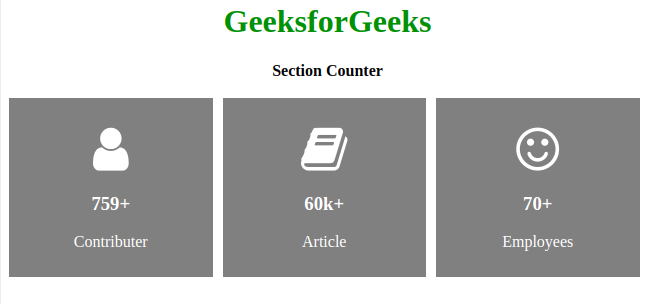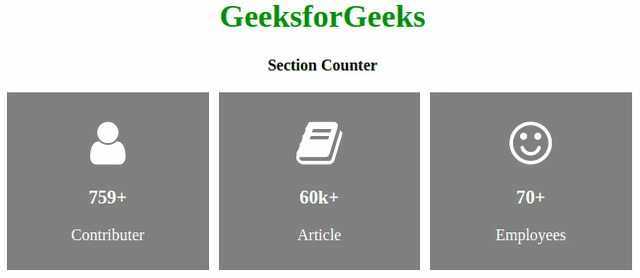# How to Create Section Counter using HTML and CSS ?Section counter is like a card and is useful for webpage footer. It contains details about the company. In this article, we will introduce some predicted data about some companies. We divide this article into two sections, in the first section we will create the normal structure then we will work on the design.

Creating the Structure: In this section, we will use simple HTML code to create three section to display few details about the company. We will use fontawesome icon to represent the functions more specifically for the user.

• CDN link for the Icons from the Font Awesome:

• HTML Code:

## html

 `` `<``html``>`   `<``head``>` `    ``<``meta` `name``=``"viewport"` `          ``content``=``"width=device-width, initial-scale=1"``>` `    ``<``link` `rel``=``"stylesheet"` `          ``href``=` `"https://cdnjs.cloudflare.com/ajax/libs/font-awesome/4.7.0/css/font-awesome.min.css"``>` ``   `<``body``>` `    ``<``h1``>GeeksforGeeks` `    ``<``strong``>Section Counter` `    ``<``br``><``br``>` `  `  `    ``<``div` `class``=``"row"``>` `        ``<``div` `class``=``"column"``>` `            ``<``div` `class``=``"card"``>` `                ``<``p``>` `                    ``<``i` `class``=``"fa fa-user"``>` `                ``` `                ``<``h3``>759+` `                ``<``p``>Contributor` `            ``` `        ``` `        ``<``div` `class``=``"column"``>` `            ``<``div` `class``=``"card"``>` `                ``<``p``>` `                  ``<``i` `class``=``"fa fa-book"``>` `                  ``` `                ``<``h3``>60k+` `                ``<``p``>Article` `            ``` `        ``` `      `  `        ``<``div` `class``=``"column"``>` `            ``<``div` `class``=``"card"``>` `                ``<``p``>` `                  ``<``i` `class``=``"fa fa-smile-o"``>` `                  ``` `                  ``` `                ``<``h3``>70+` `                ``<``p``>Employees` `            ``` `        ``` `    ``` ``   ``

Designing the Structure: We have already created the structure and here we will design the structure by using simple CSS. Also, we will add some animation to it.

• CSS Code:

## CSS

 ``

Final Solution: In this section, we will combine the above two sections in a single file then we will get the complete solution.

• Program:

## html

 `` `<``html``>`   `<``head``>` `    ``<``meta` `name``=``"viewport"` `          ``content``=``"width=device-width, initial-scale=1"``>` `    ``<``link` `rel``=``"stylesheet"` `          ``href``=` `"https://cdnjs.cloudflare.com/ajax/libs/font-awesome/4.7.0/css/font-awesome.min.css"``>    `   `      ``<``style``>` `        ``h1 {` `            ``color: green;` `        ``}` `        `  `        ``* {` `            ``box-sizing: border-box;` `        ``}` `        `  `        ``body {` `            ``text-align: center;` `        ``}` `              `  `        ``/* Float three columns */` `        ``.column {` `            ``float: left;` `            ``width: 33%;` `            ``padding: 0 5px;` `        ``}` `        `  `        ``.row {` `            ``margin: 0 -5px;` `        ``}` `              `  `        ``/* Clear floats after the columns */` `        ``.row:after {` `            ``content: "";` `            ``display: table;` `        ``}` `              `  `              `  `        ``/* Style the cards */` `        ``.card {` `            ``padding: 10px;` `            ``text-align: center;` `            ``background-color: gray;` `            ``color: white;` `        ``}` `        `  `        ``.card:hover {` `            ``transform: scale(1.1);` `            ``background-color: black;` `            ``transition-duration: 2s;` `            ``color: white;` `        ``}` `        `  `        ``.fa {` `            ``font-size: 50px;` `        ``}` `    ``` ``   `<``body``>` `    ``<``h1``>GeeksforGeeks` `    ``<``strong``>Section Counter` `    ``<``br``><``br``>` `  `  `    ``<``div` `class``=``"row"``>` `        ``<``div` `class``=``"column"``>` `            ``<``div` `class``=``"card"``>` `                ``<``p``>` `                  ``<``i` `class``=``"fa fa-user"``>` `                  ``` `                ``<``h3``>759+` `                ``<``p``>Contributor` `            ``` `        ``` `      `  `        ``<``div` `class``=``"column"``>` `            ``<``div` `class``=``"card"``>` `                ``<``p``>` `                      ``<``i` `class``=``"fa fa-book"``>` `                  ``` `                ``<``h3``>60k+` `                ``<``p``>Article` `            ``` `        ``` `      `  `        ``<``div` `class``=``"column"``>` `            ``<``div` `class``=``"card"``>` `                ``<``p``>` `                      ``<``i` `class``=``"fa fa-smile-o"``>` `                  ``` `                ``<``h3``>70+` `                ``<``p``>Employees` `            ``` `        ``` `    ``` ``   ``

Output:Whether you're preparing for your first job interview or aiming to upskill in this ever-evolving tech landscape, GeeksforGeeks Courses are your key to success. We provide top-quality content at affordable prices, all geared towards accelerating your growth in a time-bound manner. Join the millions we've already empowered, and we're here to do the same for you. Don't miss out - check it out now!

Previous
Next